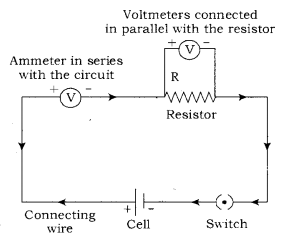# (a) State Ohm’s Law

(a) State Ohm’s Law
(b) Draw a schematic diagram of the circuit for studying ohm’s law.

(a) Ohm’s Law states that at constant temperature, the current flowing through a conductor is directly proportional to the potential difference across its ends.

(b)